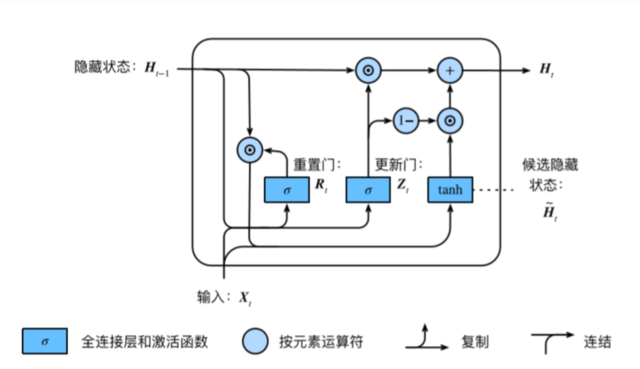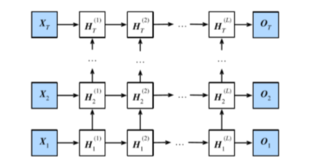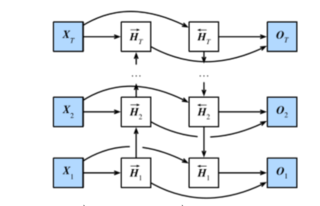# DL notes 01：RNN/LSTM/GRU

@[toc]

## 一、RNN基本结构、梯度消失和梯度爆炸的原因

$$\frac{\partial L{3}}{\partial W{X}} = \frac{\partial L{3}}{\partial O{3}} \frac{\partial O{3}}{\partial H{3}} \frac{\partial H{3}}{\partial W{X}} + \frac{\partial L{3}}{\partial O{3}} \frac{\partial O{3}}{\partial H{3}} \frac{\partial H{3}}{\partial H{2}} \frac{\partial H{2}}{\partial W{X}} + \frac{\partial L{3}}{\partial O{3}} \frac{\partial O{3}}{\partial H{3}} \frac{\partial H{3}}{\partial H{2}} \frac{\partial H{2}}{\partial H{1}} \frac{\partial H{1}}{\partial W{X}}$$

$$\frac{\partial L{3}}{\partial W{H}} = \frac{\partial L{3}}{\partial O{3}} \frac{\partial O{3}}{\partial H{3}} \frac{\partial H{3}}{\partial W{H}} + \frac{\partial L{3}}{\partial O{3}} \frac{\partial O{3}}{\partial H{3}} \frac{\partial H{3}}{\partial H{2}} \frac{\partial H{2}}{\partial W{H}} + \frac{\partial L{3}}{\partial O{3}} \frac{\partial O{3}}{\partial H{3}} \frac{\partial H{3}}{\partial H{2}} \frac{\partial H{2}}{\partial H{1}} \frac{\partial H{1}}{\partial W{H}}$$

def grad_clipping(params, theta, device):
norm = torch.tensor([0.0], device=device)
for param in params:
norm = norm.sqrt().item()
if norm > theta:
for param in params:
param.grad.data *= (theta / norm)

## 二、LSTM

LSTM主要包括以下几个结构： - 遗忘门:控制上一时间步的记忆细胞 - 输入门:控制当前时间步的输入 - 输出门:控制从记忆细胞到隐藏状态 - 记忆细胞：⼀种特殊的隐藏状态的信息的流动 三个门输出都经过诸如$sigmoid$的激活函数，映射到$(0,1)$的范围，形成门控状态。而记忆细胞则是通过$tanh$转换成$(-1,1)$的范围，这里作为记忆细胞短期依赖输出而非门控信号。

LSTM 内部主要有三个阶段：

1. 忘记阶段。这个阶段主要是对上一个节点传进来的输入进行选择性忘记。简单来说就是会 “忘记不重要的，记住重要的”。具体来说是通过计算得到的 $F{t}$ （f表示forget）来作为忘记门控，来控制上一个状态的$C{t-1}$ 哪些需要留哪些需要忘。

2. 选择记忆阶段。这个阶段将这个阶段的输入有选择性地进行“记忆”。主要是会对输入 $X_{t}$进行选择记忆。哪些重要则着重记录下来，哪些不重要，则少记一些。当前的输入内容由前面计算得到的$\widetilde{C}t$表示。而选择的门控信号则是由 $I{t}$ （i代表information）来进行控制。 >将上面两步得到的结果相加，即可得到传输给下一个状态的$C_{t}$ 。也就是上图中的第一个公式。这里也使用$tanh$起到对输入的信息进行压缩的作用。

3. 输出阶段。这个阶段将决定哪些将会被当成当前状态的输出。主要是通过 $O{t}$来进行控制的。并且还对上一阶段得到的 $C{t}$进行了放缩（通过一个tanh激活函数进行变化）。

## 三、GRU

GRU（Gate Recurrent Unit）是循环神经网络（Recurrent Neural Network, RNN）的一种。和LSTM（Long-Short Term Memory）一样，也是为了解决长期记忆和反向传播中的梯度等问题而提出来的。

GRU和LSTM在很多情况下实际表现上相差无几，那么为什么我们要使用新人GRU（2014年提出）而不是相对经受了更多考验的LSTM（1997提出）呢？其实通过代码我们就可以发现，GRU的权重参数相比LSTM减少了1/4。相比LSTM，使用GRU能够达到相当的效果，并且相比之下更容易进行训练，能够很大程度上提高训练效率，因此很多时候会更倾向于使用GRU。$$R_{t} = σ(XtW{xr} + H{t−1}W{hr} + br)$$ $$Z{t} = σ(XtW{xz} + H{t−1}W{hz} + bz)$$ $$\widetilde{H}{t-1} = Rt ⊙H{t−1}$$ $$\widetilde{H}_t = tanh(XtW{xh} + \widetilde{H}{t-1}W{hh} + b_h)$$ $$H_t = Zt⊙H{t−1} + (1−Z_t)⊙\widetilde{H}_t$$

GRU很聪明的一点就在于，我们使用了同一个门控$Z_t$ 就同时可以进行遗忘和选择记忆（LSTM则要使用多个门控）。$R_t$在GRU中被称作重置⻔有助于捕捉时间序列⾥短期的依赖关系；$Z_t$被称作更新⻔有助于捕捉时间序列⾥⻓期的依赖关系。 $Zt⊙H{t−1}$：表示对原本隐藏状态的选择性“遗忘”。这里的 $Zt$ 可以想象成遗忘门（forget gate），忘记$H{t−1}$ 维度中一些不重要的信息。 $(1−Z_t)⊙\widetilde{H}_t$： 表示对包含当前节点信息的$\widetilde{H}_t$ 进行选择性”记忆“。与上面类似，这里的$(1−Z_t)$同理会忘记 $\widetilde{H}_t$维度中的一些不重要的信息。或者，这里我们更应当看做是对$\widetilde{H}_t$ 维度中的某些信息进行选择。 $H_t = Zt⊙H{t−1} + (1−Z_t)⊙\widetilde{H}t$ ：结合上述，这一步的操作就是忘记传递下来的 $H{t−1}$ 中的某些维度信息，并加入当前节点输入的某些维度信息。 >可以看到，这里的遗忘 $Z_t$和选择$(1−Z_t)$ 是联动的。也就是说，对于传递进来的维度信息，我们会进行选择性遗忘，则遗忘了多少权重 （$Z_t$），我们就会使用包含当前输入的 $\widetilde{H}_t$ 中所对应的权重进行弥补 $(1−Z_t)$。以保持一种”恒定“状态。

## 四、深度循环神经网络$$\boldsymbol{H}_t^{(1)} = \phi(\boldsymbol{X}t \boldsymbol{W}{xh}^{(1)} + \boldsymbol{H}{t-1}^{(1)} \boldsymbol{W}{hh}^{(1)} + \boldsymbol{b}_h^{(1)})$$$$\boldsymbol{H}_t^{(\ell)} = \phi(\boldsymbol{H}t^{(\ell-1)} \boldsymbol{W}{xh}^{(\ell)} + \boldsymbol{H}{t-1}^{(\ell)} \boldsymbol{W}{hh}^{(\ell)} + \boldsymbol{b}_h^{(\ell)})$$$$\boldsymbol{O}_t = \boldsymbol{H}t^{(L)} \boldsymbol{W}{hq} + \boldsymbol{b}_q$$ 在pytorch的实现中以num_layers参数进行层数的设置和调整。

## 五、双向循环神经网络$$\overrightarrow{\boldsymbol{H}}_t = \phi(\boldsymbol{X}t \boldsymbol{W}{xh}^{(f)} + \overrightarrow{\boldsymbol{H}}{t-1} \boldsymbol{W}{hh}^{(f)} + \boldsymbol{b}_h^{(f)})$$$$\overleftarrow{\boldsymbol{H}}_t = \phi(\boldsymbol{X}t \boldsymbol{W}{xh}^{(b)} + \overleftarrow{\boldsymbol{H}}{t+1} \boldsymbol{W}{hh}^{(b)} + \boldsymbol{b}_h^{(b)})$$$$\boldsymbol{H}t=(\overrightarrow{\boldsymbol{H}}{t}, \overleftarrow{\boldsymbol{H}}_t)$$$$\boldsymbol{O}_t = \boldsymbol{H}t \boldsymbol{W}{hq} + \boldsymbol{b}_q$$ 在pytorch的实现中以bidirectional参数进行层数的设置和调整。